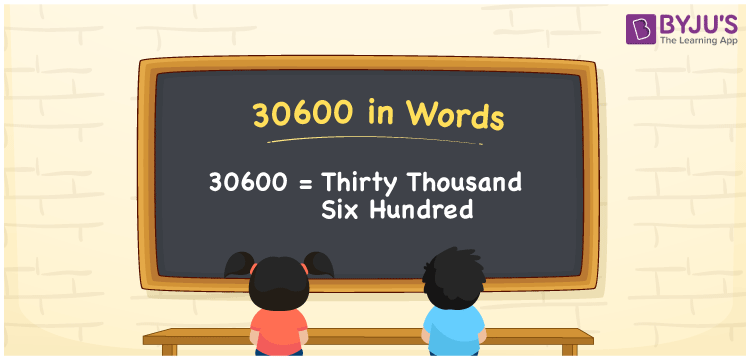# 30600 in Words

The number 30600 in words is written as Thirty thousand six hundred. We can easily convert the number 30600 into words with the help of a place value chart. Also, it is to be noted that the word form of 30600 is used to spell or express the number 30600 to others. Suppose you spotted the number 30600 on a whiteboard; you may read it as “Thirty thousand six hundred”. In the same way, we can express all cardinal numbers in their word forms.

 30600 in words Thirty thousand six hundred Thirty thousand six hundred in Numbers 30600

## 30600 in English words

Generally, we write numbers in words using the English alphabet. So, we can express 30600 in English words as “Thirty thousand six hundred”.## How to Write 30600 in Words?

Let’s learn the conversion of the number 30600 into words using a five-column place value chart since it has five digits. This is given in the table below.

 Ten thousand Thousands Hundreds Tens Ones 3 0 6 0 0

Here, ones = 0, tens = 0, hundreds = 6, thousands = 0, ten thousands = 3

By expanding these numbers, we get;

3 × Ten thousand + 0 × Thousand + 5 × Hundred + 0 × Ten + 0 × One

= 3 × 10000 + 0 × 1000 + 6 × 100 + 0 × 10 + 0 × 1

= 30000 + 600

= Thirty thousand + Six hundred

= Thirty thousand six hundred

Therefore, 30600 in words = Thirty thousand six hundred.

30600 is a natural number that is the successor of 30599 and the predecessor of 30601.

30600 in words – Thirty thousand six hundred

Is 30600 an odd number? – No

Is 30600 an even number? – Yes

Is 30600 a perfect square number? – No

Is 30600 a perfect cube number? – No

Is 30600 a prime number? – No

Is 30600 a composite number? – Yes

## Frequently Asked Questions on 30600 in Words

Q1

### How do you write 30600 in words?

We can write 30600 in words as Thirty thousand six hundred.
Q2

### How to write Rs. 30600 in words on a cheque?

On a cheque, the amount Rs. 30600 can be written in words as “Thirty thousand six hundred rupees only”.
Q3

### What is the value of thirty thousand plus six hundred in word form?

Thirty thousand plus six hundred = 30000 + 600 = 30600 = Thirty thousand six hundred Thus, the value of thirty thousand plus six hundred in word form is thirty thousand six hundred.